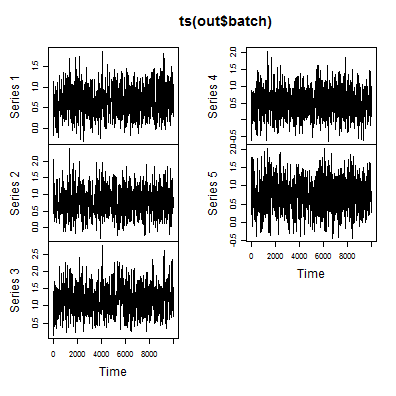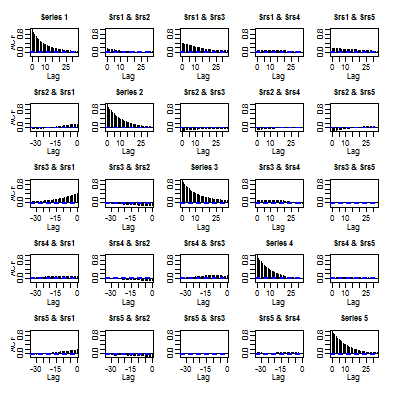MCMC案例学习

1. 问题的提出

library(mcmc)
data(logit)
out <- glm(y ~ x1 + x2 + x3 + x4, data = logit, family = binomial(), x = T)
summary(out)

Call:
glm(formula = y ~ x1 + x2 + x3 + x4, family = binomial(), data = logit,
x = T)

Deviance Residuals:
Min      1Q  Median      3Q     Max
-1.746  -0.691   0.154   0.704   2.194

Coefficients:
Estimate Std. Error z value Pr(>|z|)
(Intercept)    0.633      0.301    2.10   0.0354 *
x1             0.739      0.362    2.04   0.0410 *
x2             1.114      0.363    3.07   0.0021 **
x3             0.478      0.354    1.35   0.1766
x4             0.694      0.399    1.74   0.0817 .
---
Signif. codes:  0 '***' 0.001 '**' 0.01 '*' 0.05 '.' 0.1 ' ' 1

(Dispersion parameter for binomial family taken to be 1)

Null deviance: 137.628  on 99  degrees of freedom
Residual deviance:  87.668  on 95  degrees of freedom
AIC: 97.67

Number of Fisher Scoring iterations: 6

x <- out$x y <- out$y
lupost <- function(beta, x, y) {
eta <- as.numeric(x %*% beta)
logp <- ifelse(eta < 0, eta - log1p(exp(eta)), -log1p(exp(-eta)))
logq <- ifelse(eta < 0, -log1p(exp(eta)), -eta - log1p(exp(-eta)))
logl <- sum(logp[y == 1]) + sum(logq[y == 0])
return(logl - sum(beta^2)/8)
}

2. 开始MCMC处理

set.seed(42)
beta.init <- as.numeric(coefficients(out))
out <- metrop(lupost, beta.init, 1000, x = x, y = y)
names(out)
 "accept"       "batch"        "initial"      "final"
 "initial.seed" "final.seed"   "time"         "lud"
 "nbatch"       "blen"         "nspac"        "scale"
 "debug"

• lupost一个函数对象，即后验分布概率密度函数的对数。
• beta.init用来设定马氏链的初始状态。这里是上面广义线性模型的参数估计结果。
• nbatch = 1000是采样的样本容量，也就是马氏链的转移次数。
• x、y，是传入lupost函数中的一些参数。

metrop()函数的结果是一个list(列表)。模拟的数据存放在out$batch里。刚才的函数运行结果显示接受概率(accept)很低，所以我们调整一下proposal(建议分布)的重要参数scale(即随机游走MH算法的方差)来获得一个更合理的接受概率。什么是接受概率？我们用马氏链的方法对状态空间进行采样，那必然我们有一些值是访问不到的，接受概率就是对这种状况进行衡量的。接受概率大，说明访问越充分，接受概率小，访问就受到了一定的限制，访问不是很细致，但样本的自相关性会减弱。因此，接受概率不是越大越好，也不是越小越好。到底多大的接受概率我们认为才是合理的呢？理论显示，在五个需要估计参数的条件下，接受概率达到20%即可。我们开始尝试不同的scale值进行测试： out <- metrop(out, scale = 0.1, x = x, y = y) out$accept
 0.739
out <- metrop(out, scale = 0.3, x = x, y = y)
out$accept  0.371 out <- metrop(out, scale = 0.5, x = x, y = y) out$accept
 0.148
out <- metrop(out, scale = 0.4, x = x, y = y)
out$accept  0.209 out <- metrop(out, nbatch = 10000, x = x, y = y) out$accept
 0.2345

3. 诊断，确定批次长度

out <- metrop(out, nbatch = 10000, x = x, y = y)
out$accept  0.2332 out$time
user  system elapsed
2.40    0.00    2.41
plot(ts(out$batch))解释一下metrop()中的参数nbatch，其实与它对应的还有一个参数是blennbatch是要模拟的批数，blen是要模拟的每批的长度，blen默认为1。假如nbatch=100blen=100，那么马氏链共转移100*100=10000次. acf(out$batch)4. MCMC 的参数估计值与标准误

out <- metrop(out, nbatch = 100, blen = 100, outfun = function(z, ...) c(z,
z^2), x = x, y = y)
out$accept  0.2345 out$time
user  system elapsed
2.46    0.00    2.54

outfun函数的作用是方便构建一些统计量。对于这个问题，我们关心的是后验均值和方差。均值的计算相对简单，只需要对涉及的变量进行平均。但是方差的计算有点棘手。我们需要利用等式
$$var(X)=E(X^2)-E(X)^2$$

nrow(out$batch)  100 out$batch[1, ]
 0.6239 0.8217 1.1411 0.4686 0.7494 0.4520 0.7730 1.3696 0.3048 0.6358

out$batch[1, 1] = 0.6239是第一批样本的一阶样本矩，out$batch[1, 6] = 0.452是第一批样本的二阶样本矩，注意这里out$batch[1, 1]$^2$与out$batch[1, 6]并不相等，因为这里的每批长度(blen)是100,只有当blen=1时，它们才相等。

outfun()函数中参数…是必要的，因为函数同样需要传递其他参数(如这里的xy)到metrop()

4.1 简单均值的计算

apply(out$batch, 2, mean)  0.6712 0.7771 1.1814 0.5114 0.7729 0.5465 0.7336 1.5399 0.3878 0.7453 前五个数就是对后验参数均值的蒙特卡洛估计值，紧随其后的五个数是对后验参数二阶矩的估计值。通过下面的程序，得到参数的方差估计. foo <- apply(out$batch, 2, mean)
mu <- foo[1:5]
sigmasq <- foo[6:10] - mu^2
mu
 0.6712 0.7771 1.1814 0.5114 0.7729

(注：方差和标准误是两个不同的概念。方差是一个参数估计，而标准误是对参数估计好坏评价的度量。)

mu.muce <- apply(out$batch[, 1:5], 2, sd)/sqrt(out$nbatch)
mu.muce
= NA  0.01367 0.01518 0.01785 0.01585 0.01622

4.2 均值的函数

$$g(u,v)=v-u^{2}$$
$$\Delta g(u,v)=\Delta v-2u\Delta u$$

$$\frac{1}{n_{batch}} \displaystyle \sum_{i=1}^{n_{batch}}[(v_{i} – \overline v)-2\overline u (u_{i} – \overline u)]^2$$

u <- out$batch[, 1:5] v <- out$batch[, 6:10]
ubar <- apply(u, 2, mean)
vbar <- apply(v, 2, mean)
deltau <- sweep(u, 2, ubar)
deltav <- sweep(v, 2, vbar)
foo <- sweep(deltau, 2, ubar, "*")
sigmasq.mcse <- sqrt(apply((deltav - 2 * foo)^2, 2, mean)/out$nbatch) sigmasq.mcse  0.004687 0.008586 0.007195 0.007666 0.007714 这五个值就是后验方差的蒙特卡洛标准误(MCSE)。 4.3 均值函数的函数 如果我们对后验标准差也感兴趣的话，也可以通过delta method计算它的标准误，程序如下 sigma <- sqrt(sigmasq) sigma.mcse <- sigmasq.mcse/(2 * sigma) sigma.mcse  0.007565 0.011923 0.009470 0.010789 0.010029 5. 最后的运行 问题已经解决。现在唯一需要改进的就是提高结果的精确度。(试题要求“你的马尔科夫链采样器必须足够长以保证参数估计的标准误低于0.01”)。取模拟批次为500，每批长度为400，运行如下： out <- metrop(out, nbatch = 500, blen = 400, x = x, y = y) out$accept
 0.2352
out$time user system elapsed 50.40 0.01 51.01 (显然，由于模拟的次数增大，程序运行时间变长，当然不同运算速度的计算机可能显示结果并不一样。下面进行均值和方差的估计。) foo <- apply(out$batch, 2, mean)
mu <- foo[1:5]
sigmasq <- foo[6:10] - mu^2
mu
 0.6636 0.7961 1.1712 0.5075 0.7241

mu.muce <- apply(out$batch[, 1:5], 2, sd)/sqrt(out$nbatch)
mu.muce
 0.002972 0.003611 0.003828 0.003604 0.004242

u <- out$batch[, 1:5] v <- out$batch[, 6:10]
ubar <- apply(u, 2, mean)
vbar <- apply(v, 2, mean)
deltau <- sweep(u, 2, ubar)
deltav <- sweep(v, 2, vbar)
foo <- sweep(deltau, 2, ubar, "*")
sigmasq.mcse <- sqrt(apply((deltav - 2 * foo)^2, 2, mean)/out\$nbatch)
sigmasq.mcse
 0.001062 0.001718 0.001538 0.001574 0.002007

sigma <- sqrt(sigmasq)
sigma.mcse <- sigmasq.mcse/(2 * sigma)
sigma.mcse
 0.001752 0.002355 0.002116 0.002192 0.002509

• 后验均值估计：
library(xtable)
data1 <- rbind(mu, mu.muce)
colnames(data1) <- c("constant", "x1", "x2", "x3", "x4")
rownames(data1) <- c("estimate", "MCSE")
data1.table <- xtable(data1, digits = 5)
print(data1.table, type = "html")
constant x1 x2 x3 x4
estimate 0.66365 0.79608 1.17118 0.50755 0.72414
MCSE 0.00297 0.00361 0.00383 0.00360 0.00424
• 后验方差估计
data2 <- rbind(sigmasq, sigmasq.mcse)
colnames(data2) <- c("constant", "x1", "x2", "x3", "x4")
rownames(data2) <- c("estimate", "MCSE")
data2.table <- xtable(data2, digits = 5)
print(data2.table, type = "html")
constant x1 x2 x3 x4
estimate 0.09195 0.13302 0.13200 0.12890 0.15997
MCSE 0.00106 0.00172 0.00154 0.00157 0.00201
• 后验标准差估计
data3 <- rbind(sigma, sigma.mcse)
colnames(data3) <- c("constant", "x1", "x2", "x3", "x4")
rownames(data3) <- c("estimate", "MCSE")
data3.table <- xtable(data3, digits = 5)
print(data3.table, type = "html")
constant x1 x2 x3 x4
estimate 0.30323 0.36472 0.36332 0.35903 0.39997
MCSE 0.00175 0.00236 0.00212 0.00219 0.00251《MCMC案例学习》有15个想法

1.丁鹏说道：

拟合bayesian GLM，请用MCMCpack。

在现实中，一般不会这样直接的使用metropolis。MCMCpack的算法是正态逼近后验，用来作为metropolis的proposal。

如果直接暴力的使用metropolis，大部分时候效果奇差，尤其是X之间的相关性强的时候，mcmc的ACF太大，mixing效果不好。

1.akward说道：

请问，我的代码：
library(MCMCpack)
out1 <- MCMCprobit(y~et+TFP+Size+Wage+Age+Mid,data=export_data,burnin = 5000, mcmc = 10000,
b0 = c(0,0,0,0,0,0), B0 = c(100,100,100,100,100,100), marginal.likelihood="Chib95")
plot(out2)
summary(out2)

参数估计结果里有个sigma是什么？
这个是用来衡量什么的？
模型估计的好坏看什么？有拟合优度指标没？

2.陈宇说道：

大神，如果我只是相对均匀分布 和 正态分布进行采样，能用mcmc方法嘛

2.gaolei说道：

的确，处理实际问题直接使用这个函数确实不妥。其实，从贝叶斯角度解决广义线性模型，也有这样的包和函数，直接来做，比如，arm 包中的bayesglm(),与glm的使用差不多，不过其实质还是mcmc。处理各种模型，使用贝叶斯方法很普遍，参见（CRAN Task View: Bayesian Inference）。这里只是向初学者展示一下mcmc的使用过程。

1.陈宇说道：

大神，如果我只是相对 均匀分布 和 正态分布进行采样，您觉得可行嘛？那建议分布就是正态分布本身嘛？

3.Lu.M说道：

太好了，虚心学习。二位继续努力。

4.白日梦说道：

能不能在开篇的时候多讲讲建模原理和步骤, 一上来就是R代码, R基础不好的人看的不是很明白啊.

1.谢益辉说道：

这本来就是R包的文档，不可避免要上R。一篇文章不可能面面俱到，更多的功课还得你自己去做，况且无代码无真相啊。

2.gaolei说道：

一块是贝叶斯推断，一块是MCMC算法，看一下这方面的文档就可以了

5.zhudan说道：

虚心学习！

6.xwy说道：

这应该是对mcmc package demo的翻译，值得学习

7.十八学士说道：

如果其中有个参数有限制，比如非负，可以让这个参数先验分布为一个对数正态分布吗？

1.lei_gao说道：

没错，用对数正态分布作为其先验分布，能把参数限制在正数上。

8.第九街TEUFEL说道：

请问，有没有人能告诉我，metrop（lupost, beta.init, 1000, x = x, y = y)，这个写法其中x=x，y=y是定义lupost的参数x，y为样本x，y么？假设lupost的样本变量只有y，样本信息也只有一个变量y，此处应该怎样写呢？